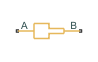# Sudden Area Change (TL)

Sudden expansion or contraction in flow area

•Libraries:
Simscape / Fluids / Thermal Liquid / Pipes & Fittings

## Description

The Sudden Area Change (TL) block models the minor pressure losses due to a sudden change in flow cross-sectional area. The area change is a contraction from port A to port B and an expansion from port B to port A. This component is adiabatic. It does not exchange heat with its surroundings.

Sudden Area Change SchematicThe pressure drop across a sudden expansion is primarily due to turbulence mixing in the expansion zone. Across a sudden contraction, it is primarily due to flow detachment at the contraction zone entrance. The figure shows the expansion and contraction zones of the sudden area change.### Mass Balance

The mass conservation equation in the sudden area change is

`${\stackrel{˙}{m}}_{A}+{\stackrel{˙}{m}}_{B}=0,$`

where:

• ${\stackrel{˙}{m}}_{A}$ and ${\stackrel{˙}{m}}_{B}$ are the mass flow rates into the sudden area change through ports A and B.

### Momentum Balance

The momentum conservation equation in the sudden area change is

`${p}_{A}-{p}_{B}=\frac{{\stackrel{˙}{m}}^{2}}{2\rho }\left(\frac{1}{{S}_{B}^{2}}-\frac{1}{{S}_{A}^{2}}\right)+{\varphi }_{Loss},$`

where:

• pA and pB are the pressures at ports A and B.

• $\stackrel{˙}{m}$ is the average mass flow rate.

• ρ is the average fluid density.

• SA and SB are the flow cross-sectional areas at ports A and B.

• ΦLoss is the mechanical energy loss due to the sudden area change.

The mechanical energy loss is

`${\varphi }_{Loss}={K}_{Loss}\frac{{\stackrel{˙}{m}}^{2}}{2\rho {S}_{B}^{2}},$`

where:

• KLoss is the loss coefficient.

If the Loss coefficient specification parameter is set to `Semi-empirical formulation`, the loss coefficient for a sudden expansion is computed as

`${K}_{Loss}={K}_{e}{\left(1-\frac{{S}_{B}}{{S}_{A}}\right)}^{2},$`

while for a sudden contraction it is computed as

`${K}_{Loss}=\frac{{K}_{c}}{2}\left(1-\frac{{S}_{B}}{{S}_{A}}\right),$`

where:

• Ke is the correction factor in the expansion zone.

• Kc is the correction factor in the contraction zone.

In the transition zone between sudden expansion and sudden contraction behavior, the loss coefficient is smoothed through a cubic polynomial function:

`${K}_{Loss}={K}_{e}{\left(1-\frac{{S}_{B}}{{S}_{A}}\right)}^{2}+\lambda \left[\frac{{K}_{c}}{2}\left(1-\frac{{S}_{B}}{{S}_{A}}\right)-{K}_{e}{\left(1-\frac{{S}_{B}}{{S}_{A}}\right)}^{2}\right],$`

where

`$\lambda =3{\overline{\stackrel{˙}{m}}}^{2}-2{\overline{\stackrel{˙}{m}}}^{3},$`

and

`${\stackrel{˙}{m}}_{Cr}={\mathrm{Re}}_{Cr}\sqrt{\frac{\pi }{4}{S}_{B}\mu .}$`

If the Loss coefficient specification parameter is set to ```Tabulated data — Loss coefficient vs. Reynolds number```, the block obtains the loss coefficient from tabular data provided as a function of the Reynolds number.

### Energy Balance

The energy conservation equation in the sudden area change is

`${\varphi }_{A}+{\varphi }_{B}=0,$`

where:

• ΦA and ΦB are the energy flow rates into the sudden area change through ports A and B.

## Assumptions and Limitations

• The flow is incompressible. The fluid density is assumed constant in the sudden area change.

## Ports

### Conserving

expand all

Fluid entry or exit point to the area change section.

Fluid entry or exit point to the area change section.

## Parameters

expand all

Parameterization for calculating the loss coefficient due to the sudden area change. Select `Semi-empirical formulation` to automatically compute the loss coefficient from the cross-sectional areas at ports A and B. Select ```Tabulated data - Loss coefficient vs. Reynolds number``` to specify a 1-D lookup table for the loss coefficient with respect to the flow Reynolds number.

Area normal to the direction of flow at inlet A. This value must be greater than the cross-sectional area at B.

Area normal to the direction of flow at B. This value must be smaller than the cross-sectional area at A.

Reynolds number at which flow transitions between laminar and turbulent regimes in the contraction portion of the sudden area change. This parameter is visible only when the Loss coefficient specification parameter is set to ```Semi-empirical formulation```.

Scaling factor for adjusting the loss coefficient value in the contraction portion of the sudden area change. The block multiplies the loss coefficient factor calculated from the semi-empirical expression by this factor. This parameter is visible only when the Loss coefficient specification parameter is set to ```Semi-empirical formulation```.

Scaling factor for adjusting the loss coefficient value in the expansion portion of the sudden area change. The block multiplies the loss coefficient factor calculated from the semi-empirical expression by this factor. This parameter is visible only when the Loss coefficient specification parameter is set to ```Semi-empirical formulation```.

Vector of Reynolds numbers for the tabular parameterization of loss coefficients. You specify the Contraction loss coefficient vector and Expansion loss coefficient vector parameters at these Reynolds numbers.

This parameter is visible only when the Loss coefficient specification parameter is set to ```Tabulated data - Loss coefficient vs. Reynolds number```.

Vector of loss coefficients for the contraction portion of the area change. Specify the loss coefficients at the Reynolds numbers in the Reynolds number vector parameter. The block uses the Reynolds number and loss coefficient vectors to construct a 1-D lookup table.

This parameter is visible only when the Loss coefficient specification parameter is set to ```Tabulated data — Loss coefficient vs. Reynolds number```.

Vector of loss coefficients for the expansion portion of the area change. Specify the loss coefficients at the Reynolds numbers in the Reynolds number vector parameter. The block uses the Reynolds number and loss coefficient vectors to construct a 1-D lookup table.

This parameter is visible only when the Loss coefficient specification parameter is set to ```Tabulated data — Loss coefficient vs. Reynolds number```.

## Version History

Introduced in R2016a